#Function Repository Resource:

# NFourierSequenceTransform

Find a numerical approximation to the Fourier sequence transform

Contributed by: Wolfram Research
 ResourceFunction["NFourierSequenceTransform"][expr,n,ω] gives a numerical approximation to the Fourier sequence transform of expr evaluated at the numerical value ω, where expr is a function of n.

## Details and Options

Fourier sequence transform is also known as discrete-time Fourier transform (DTFT).
ResourceFunction["NFourierSequenceTransform"][expr,n,ω] takes a sequence whose nth term is given by expr.
The numerical approximation to the Fourier sequence transform of expr is by default defined to be NSum[expr-nω,{n,-∞,}].
Different choices for the definition of the Fourier sequence transform can be specified using the option FourierParameters.
With the setting FourierParameters{a,b}, the Fourier sequence transform computed by ResourceFunction["NFourierSequenceTransform"] is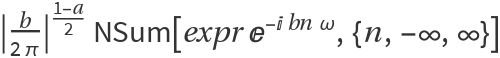.
The parameter b in the setting FourierParameters{a,b} must be numeric.
In addition to the option FourierParameters, ResourceFunction["NFourierSequenceTransform"] can also accept the options available to NSum. These options are passed directly to NSum.

## Examples

### Basic Examples

Different definitions for numerical approximation of a Fourier sequence transform:

 In:=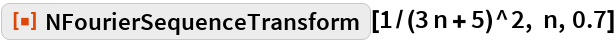Out=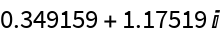In:=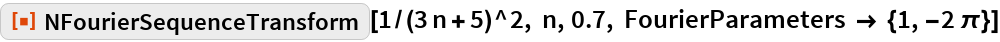Out=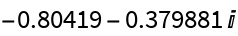Compare with the answer from symbolic evaluation with FourierSequenceTransform:

 In:=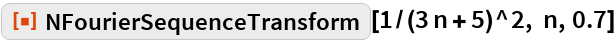Out=In:=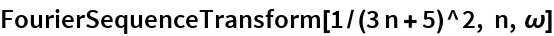Out=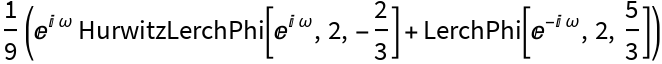In:=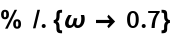Out=## Requirements

Wolfram Language 11.3 (March 2018) or above